Test: Quantitative Techniques- 3

# Test: Quantitative Techniques- 3

Test Description

## 20 Questions MCQ Test Mock Test Series for CLAT | Test: Quantitative Techniques- 3

Test: Quantitative Techniques- 3 for Banking Exams 2023 is part of Mock Test Series for CLAT preparation. The Test: Quantitative Techniques- 3 questions and answers have been prepared according to the Banking Exams exam syllabus.The Test: Quantitative Techniques- 3 MCQs are made for Banking Exams 2023 Exam. Find important definitions, questions, notes, meanings, examples, exercises, MCQs and online tests for Test: Quantitative Techniques- 3 below.
Solutions of Test: Quantitative Techniques- 3 questions in English are available as part of our Mock Test Series for CLAT for Banking Exams & Test: Quantitative Techniques- 3 solutions in Hindi for Mock Test Series for CLAT course. Download more important topics, notes, lectures and mock test series for Banking Exams Exam by signing up for free. Attempt Test: Quantitative Techniques- 3 | 20 questions in 30 minutes | Mock test for Banking Exams preparation | Free important questions MCQ to study Mock Test Series for CLAT for Banking Exams Exam | Download free PDF with solutions
 1 Crore+ students have signed up on EduRev. Have you?
Test: Quantitative Techniques- 3 - Question 1

### If one-ninth of a certain number exceeds its one-tenth by 4, the number is

Detailed Solution for Test: Quantitative Techniques- 3 - Question 1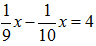x = 360

Test: Quantitative Techniques- 3 - Question 2

### A coin is tossed thrice. The probability that exactly two heads show up is

Detailed Solution for Test: Quantitative Techniques- 3 - Question 2

As we know that A coin has two faces head and tail.
Total (Exhaustive) number of cases = 23 = 8
Possible outcomes = (HHH, HHT, HTH, THH, HTT, TTH, THT, TTT)
Favourable number of cases = 3

Required probability = 3/8

Test: Quantitative Techniques- 3 - Question 3

### The angles of a triangle are in the ratio 3 : 4 : 5. The measure of the largest angle of the triangle is

Detailed Solution for Test: Quantitative Techniques- 3 - Question 3

Let the angles be x°
Then,
We know sum of all interior angles of a triangle is 180° so According to problem we must have to find out the value of x° to be correct.

3x + 4x + 5x = 180
⇒ 12x = 180
⇒    x = 180/12
⇒    x = 15

Therefore x° = 15

Here the largest in ratio is 5 so we can write that 5x is the largest angle.

5x = 5×15 = 75°

Test: Quantitative Techniques- 3 - Question 4

The sum of three numbers is 98. If the ratio of the first to the second is 2 : 3 and that of the second to the third is 5 : 8, then the second number is

Detailed Solution for Test: Quantitative Techniques- 3 - Question 4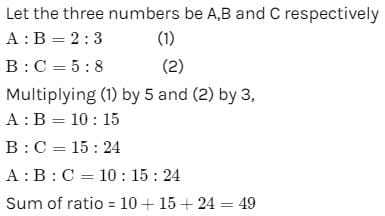So second no. (B) =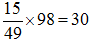Test: Quantitative Techniques- 3 - Question 5

If the circumference of a circle is reduced by 50%, its area will be reduced by

Detailed Solution for Test: Quantitative Techniques- 3 - Question 5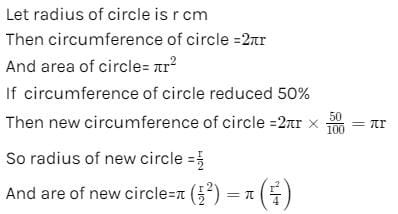Reduction in area = πr2−πr2​/4 =3πr2/4

% reduction = 3πr2/(4 x πr2) x 100

= 75%

Test: Quantitative Techniques- 3 - Question 6

12 persons can do a piece of work in 4 days. How many persons are required to complete 8 times the work in half the time?

Detailed Solution for Test: Quantitative Techniques- 3 - Question 6

The work requires 12 persons x 4 days = 48 person-days.

A work that is 8 times that size will need 48x*8 = 384 person-days.

Now if the work is to be done in 4/2 days or 2 days, we need

384/2 = 192 persons.

Test: Quantitative Techniques- 3 - Question 7

Two pipes can fill a tank in 15 hours and 20 hours respectively, while the third can empty it in 30 hours. If all the pipes are opened simultaneously, the empty tank will be filled in

Detailed Solution for Test: Quantitative Techniques- 3 - Question 7

Let the capacity of the tank be 60 units (LCM of 15, 20 & 30).
Pipe A fills = 60/15​=4 units / hour
Pipe B fills = 60​/20=3 units / hour
Pipe A fills = 60/20​=2 units / hour
(A + B + C)'s together per hour work = (4+3−2)=5 units/hour
Time taken by all three to fill an empty tank = 60/5​=12 hours.

Test: Quantitative Techniques- 3 - Question 8

The next number of the sequence 3, 5, 9, 17, 33, …….is

Detailed Solution for Test: Quantitative Techniques- 3 - Question 8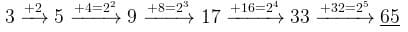Test: Quantitative Techniques- 3 - Question 9

The cost price of 8 articles is equal to the selling price of 9 articles. The profit or loss per cent in the transaction is

Detailed Solution for Test: Quantitative Techniques- 3 - Question 9

Let the C.P. of each article be 1 Rs.

∴ CP of 9 article = 9 Rs.

∴ SP of 9 article = 8 Rs.

∴ Loss = 1 Rs.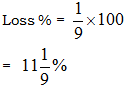Test: Quantitative Techniques- 3 - Question 10

If 78*3945 is divisible by 11. where * is a digit, then * is equal to

Detailed Solution for Test: Quantitative Techniques- 3 - Question 10

We'll apply the divisiblity rule for 11

Odd place: 7+∗+9+5=21+∗

Even place: 8+3+4=15

(21 + *) - (15) = either 11 or 0

(21 + *) - 15 = 11

21 + * = 26

* = 5

Test: Quantitative Techniques- 3 - Question 11

In a 45 litres mixture of milk and water, the ratio of the milk to water is 2 : 1. When some quantity of water is added to the mixture, this ratio becomes 1 : 2. The quantity of water added is

Detailed Solution for Test: Quantitative Techniques- 3 - Question 11

Milk : water = 2 : 1

let ratio be 2a and a

so, 2a + a = 45

a = 15

∴ Milk=2×15=30 litres

Water=1×15=15 litres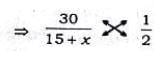60 = 15 + x

x = 45 litres

Test: Quantitative Techniques- 3 - Question 12

A and B together can do a piece of work in 10 days. A alone can do it in 30 days. The time in which B alone can do it is

Detailed Solution for Test: Quantitative Techniques- 3 - Question 12

(A+B)’s 1 day’s work = 1/10
A’s 1 day’s work = 1/30
∴ B’s 1 day’s work = 1/10 - 1/30
=2/30 = 1/15

Hence B can alone complete the work in 15 days.

Test: Quantitative Techniques- 3 - Question 13

If the price of tea is increased by 20%, by how much per cent the consumption of tea be reduced so that there is no increase in the expenditure on it?

Detailed Solution for Test: Quantitative Techniques- 3 - Question 13

Let the original price of the tea be Rs 100.
The price of the tea is increased by 20%.
Increased price of the tea = 100 + (20% of 100) = 100 + 20 = Rs 120.
Increase in price = 120 - 100 = Rs 20
Tea of cost Rs 20 is to decreased out of the increased cost of Rs 120.
Percentage decrease = (20/120) x 100 = 100/6 =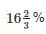Test: Quantitative Techniques- 3 - Question 14

The smallest 4-digit number, which is a perfect square, is

Detailed Solution for Test: Quantitative Techniques- 3 - Question 14

312=961

322=1024

332=1089

342=1156

Least number of four digits = 1000.

(32)2 is more than 1000 by 24.

So, the least number to be added to 1000 is 24.

1000 + 24 = 1024

Therefore, the smallest four digit number which is a perfect square is 1024.

Test: Quantitative Techniques- 3 - Question 15

The difference between a single discount of 30% on Rs. 550 and two successive discounts of 20% and 10% on the same amount is

Detailed Solution for Test: Quantitative Techniques- 3 - Question 15

Ist Discount = 30%

Net two successive Discount =

20+10−(20×10)/100
= 2%
So difference = 2% of 550 = Rs 11

Test: Quantitative Techniques- 3 - Question 16

A dealer makes a profit of 20% even after giving a 10% discount on the advertised price of a scooter. If he makes a profit of Rs. 7500 on the sale of the scooter, the advertised price was

Detailed Solution for Test: Quantitative Techniques- 3 - Question 16

According to question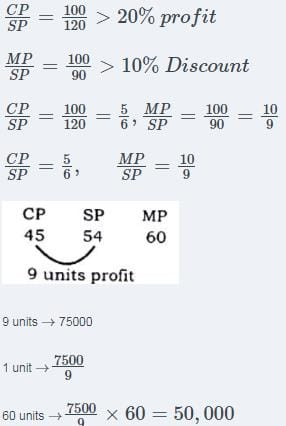MP = 50,000

Test: Quantitative Techniques- 3 - Question 17

A person standing on a railway platform noticed that a train took 21 seconds to completely pass through the platform which was 84 m long and it took 9 seconds in passing him. The speed of the train was

Detailed Solution for Test: Quantitative Techniques- 3 - Question 17

Let the length of the train be x metres

Then Speed of the train in passing through the platform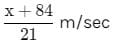and speed of the train in passing the man =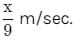Since both the speeds are the same,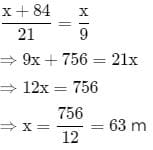∴ speed of the train =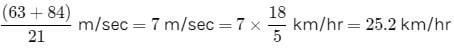Test: Quantitative Techniques- 3 - Question 18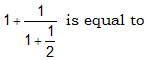Detailed Solution for Test: Quantitative Techniques- 3 - Question 18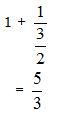Test: Quantitative Techniques- 3 - Question 19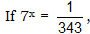then the value of x is

Detailed Solution for Test: Quantitative Techniques- 3 - Question 19

7x = 1/343

7x = 1/(73)

7x = 7(- 3)

x = - 3

Test: Quantitative Techniques- 3 - Question 20

The average of runs scored by a player in 10 innings is 50. How many runs should he score in the 11th innings so that his average is increased by 2 runs?

Detailed Solution for Test: Quantitative Techniques- 3 - Question 20

Given, The average of runs scored by a player in 10 innings = 50
Let the number of runs scored in 11th innings be n.
According to question ,
∴ 10 × 50 + n = 11 × 52
⇒ 500 + n = 572
⇒ n = 572 – 500 = 72 runs

## Mock Test Series for CLAT

2 videos|9 docs|77 tests
 Use Code STAYHOME200 and get INR 200 additional OFF Use Coupon Code
Information about Test: Quantitative Techniques- 3 Page
In this test you can find the Exam questions for Test: Quantitative Techniques- 3 solved & explained in the simplest way possible. Besides giving Questions and answers for Test: Quantitative Techniques- 3, EduRev gives you an ample number of Online tests for practice

## Mock Test Series for CLAT

2 videos|9 docs|77 tests